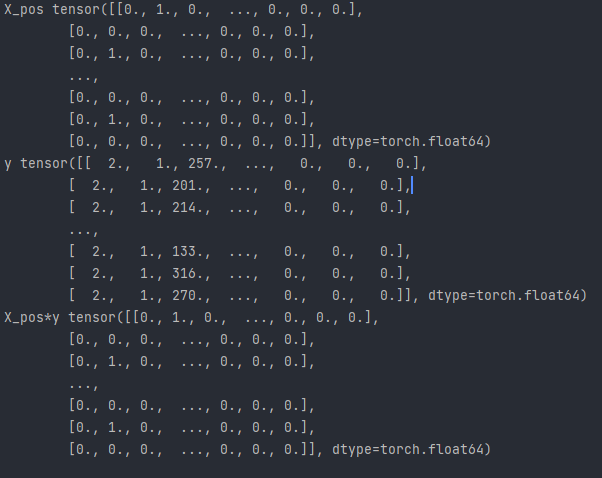# Multiplying two tensors returns first tensor

Hello,

I’m trying to multiply two tensors to mask out certain values.

``````a = torch.tensor([[1,2],[3,4]])
b = torch.tensor([[1,0],[0,1]])
c = a*b
``````

Where c should return tensor([[1, 0],[0, 4]]). This works perfectly in my python notebook.

However, in my train loop, I’m multiplying X_pos * y which outputs the X_pos tensor rather than the multiplication of the two tensors.

``````for batch in dataloader:
X, y = batch[:], batch[:]

X_pos = X[:,1,:]
X,y = X[:,0,:],y[:,0,:]

sequence_length = y.size(1)
print(X_pos.size(),X_pos)
print(y.size(),y)
print(X_pos*y)

pred = pred.permute(1,2,0)

loss = loss_fn(pred, y.type(torch.LongTensor).to(self.device))Your current screenshot shows the expected output since the values at `y` where `x` contains ones are also ones.